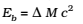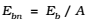As, some mass disappears in the formation of a nucleus in the form of mass defect. This loss in mass reappears in the form of energy called binding energy.

In other word, binding energy is the energy which should be supplied to the nucleus in order to break it up into its constituent particles.

### Binding energy per Nucleon

It is the ratio of the binding energy of a nucleus to the number of the nucleons.

Binding energy per nucleon = (Total binding energy) / (Number of nucleon)

For example, let us consider; a nucleus which has 8 neutrons and 8 protons.

We have
Mass of 8 neutrons = 8 × 1.00866 u
Mass of 8 protons = 8 × 1.00727 u
Mass of 8 electrons = 8 × 0.00055 u

Therefore the expected mass of nucleus = 8×2.01593 u = 16.12744 u.

The atomic mass of found from mass spectroscopy experiments is seen to be 15.99493 u. Subtracting the mass of 8 electrons (8 × 0.00055 u) from this, we get the experimental mass of nucleus to be 15.99053 u.
Thus, we find that the mass of the 16 😯 nucleus is less than the total mass of its constituents by 0.13691u. The difference in mass of a nucleus and its constituents, ΔM, is called the mass defect and is given byThis energy required Eb is related to the mass defect byIf a certain number of neutrons and protons are brought together to form a nucleus of a certain charge and mass, an energy Eb will be released in the process. The energy Eb is called the binding energy of the nucleus.

A more useful measure of the binding between the constituents of the nucleus is the binding energy per nucleon Ebn which is the ratio of the binding energy Eb of a nucleus to the number of the nucleons, A, in that nucleus:### Binding Energy Curve and its Features

Binding energy per nucleon as the average energy per nucleon needed to separate a nucleus into its individual nucleons.

Binding energy curve is a plot of the binding energy per nucleon versus the mass number for large nuclei.